## 大眾Polo Plus，配置太低，買它靠情懷？

2019年07月04日 18:20 來源：車行天下 超過：14632次關注

中國市場其實是沒有什么好的合資小型車的。因為小型車受制于成本的原因，通常價格沒有辦法賣得很高，所以對于合資品牌來說利潤太低了。目前市面上的小型車，主要是自主品牌在研發和生產，合資品牌是不涉足的，我們現在能夠脫口而出的合資小型車，除了現代和起亞這樣的合資品牌以外，就是大眾Polo Plus和本田飛度了，這兩款車是目前市面上關注度最高的，而且是品質非常好的合資小型車。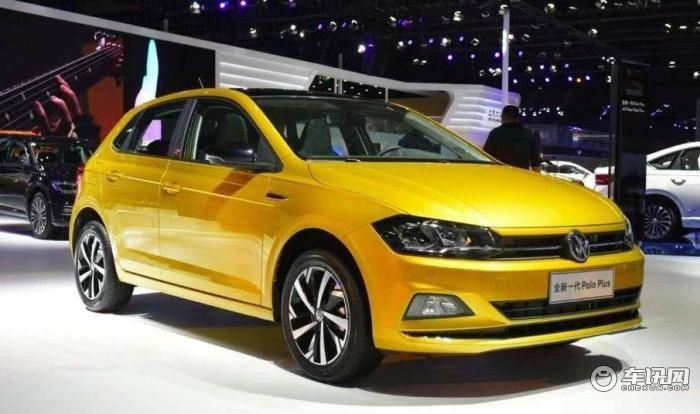隨著大眾Polo的換代，在市場上引起了非常大的熱議，因為這款車價格實在是太貴了，作為一個小型車，大眾Polo Plus的價格是9.99~12.59萬元。最低配的9.99萬元是一款手動擋的車型，并不是走量的車型，而自動擋的車型最低配也要10.99萬元起，接近11萬的價格了。而11萬的價格消費者能夠買到什么配置呢?可以說是非常非常寒酸的。因此它也就成了目前市面上性價比最差的合資小型車。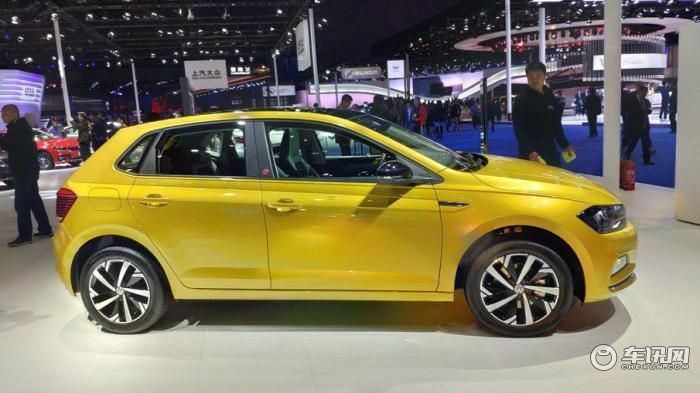不過由于大眾Polo Plus的定位以及它時尚的造型設計，還是能夠受到很多一線城市，年輕消費者的青睞，滿足他們城市代步的基本需求。不過付出的代價有點大，因為相同的價格可以買到更好的緊湊型車。而如果消費者喜歡Polo Plus這樣尺寸不大的車，就不得不接受它昂貴的價格。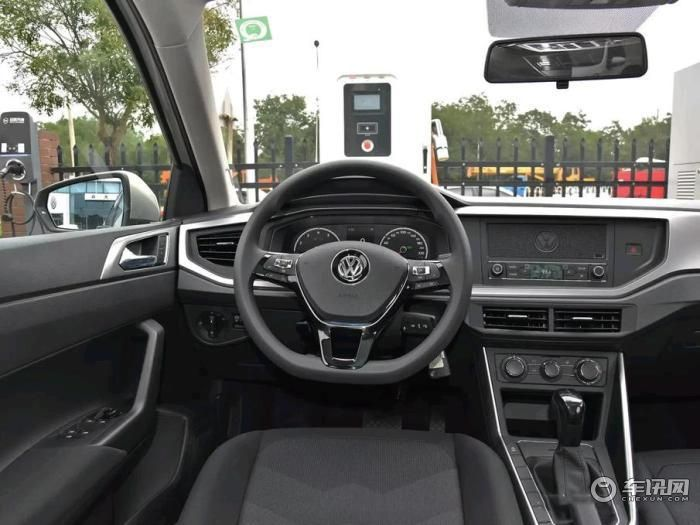6.5寸中控屏幕，11.59萬起

大眾Polo Plus在中低配車型上使用的是6.5英寸的中控屏幕，但是要11.59萬元起的價格才可以買到。其實由于大眾MQB平臺的通用性，中控大屏幕升級的成本也很低，大概也就五六百塊錢，不過對于廠商來說500塊錢就是很大的一個成本了，大家可以想一下，如果一年賣10萬輛，每輛車500塊錢成本，那么10萬輛就是5000萬元。

但是對于消費者來說實在難以接受，就是11.59萬元買不了一個帶中控屏的車。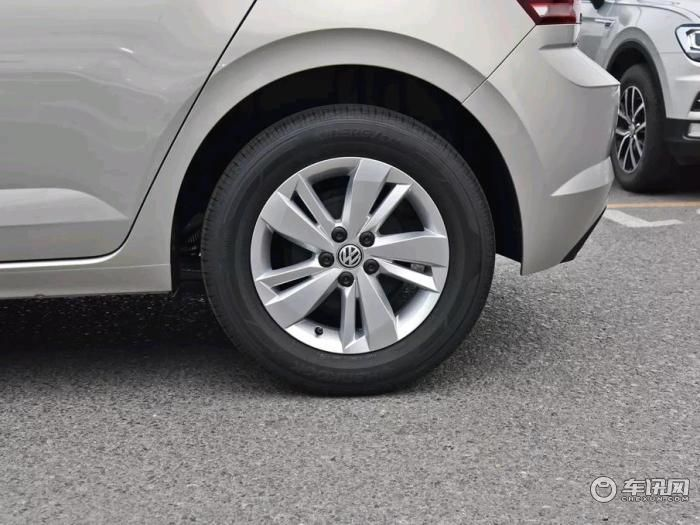后輪盤式剎車，14.39萬起

鼓式剎車和盤式剎車，是有很明顯的區別的，兩者并沒有好壞之分，通常載重量比較大的車用鼓式剎車，因為它的制動效果好，但缺點是有熱衰減而且不防水，通常對于家用轎車來說，用盤式剎車是最為普遍的。因為家用轎車重量輕，在同樣的制動效果的前提下，盤式剎車有更好的散熱效果，而且不怕水。

從成本上，鼓式剎車成本低，而Polo Plus只有頂配車型上售價14.39萬元的版本上使用了盤式剎車。可以說是很摳門了。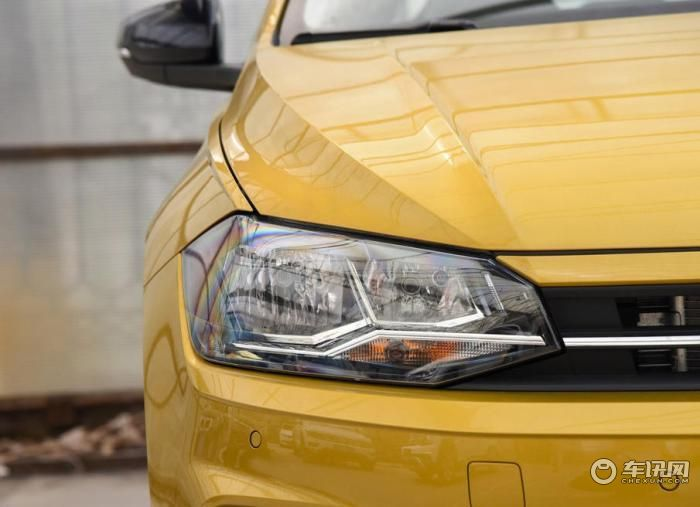LED大燈，全系沒有，頂配可以選裝

目前在國產轎車上使用LED燈已經非常非常普遍了，因為它的亮度更高，而且更加的節能，使用壽命也更長。但是Polo Plus全系都是鹵素大燈，只有最頂配的車型，消費者才有權利選裝LED大燈。如果你買的不是頂配，那你選裝也沒有辦法選，只能去后期自己改裝，而改裝的成本，其實也并不高，在電商平臺上花幾千塊錢就可以升級成LED大燈了。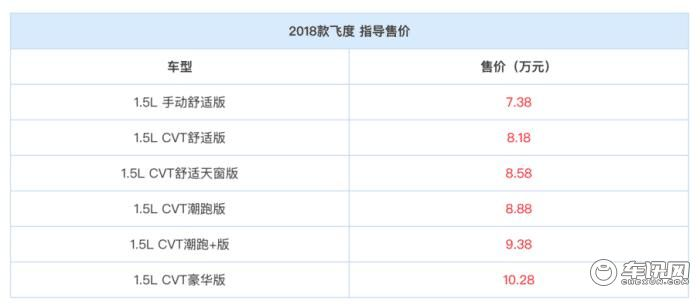Polo Plus的高價是給自己留下了降價區間?

很多人說Polo Plus的高價格，是給自己留下了降價空間。其實我并不這么認為，因為我們看一下飛度，相比起大眾來說，飛度的價格可以說是非常厚道的。起步價是7.38萬元，最頂配的價格是10.28萬元。而且目前市面上飛度也有一些優惠，所以我并不認為Polo Plus的高價格是給自己留下的降價空間，而是廠商為了賺更多的利潤，故意的把價格定得非常高，本來也沒想賣的便宜。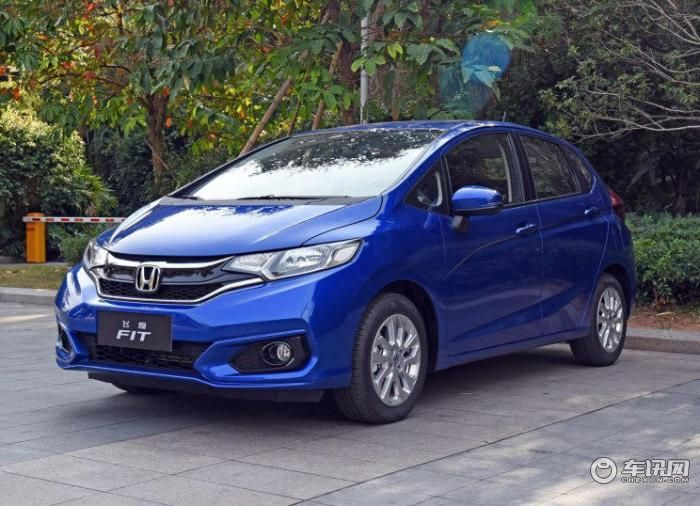同級別的合資品牌車型配置不如自主品牌的車型高，是可以理解的，因為自主品牌一直是以配置的豐富程度來吸引消費者的，不過Polo Plus是一款小型車的定位，而且還賣十幾萬的價格，配置還如此之低，真的是目前車市比較罕見的情況。所以也更加凸顯了飛度的性價比優勢。

0-500 字已有評論 0條 查看評論>>

### 熱門標簽

?
• 快速找車
• 選擇品牌
• 選擇品牌
• A  奧迪
• A  阿斯頓·馬丁
• A  阿爾法·羅密歐
• B  寶沃
• B  布加迪
• B  巴博斯
• B  保時捷
• B  賓利
• B  奔馳
• B  寶馬
• B  本田
• B  別克
• B  標致
• B  比亞迪
• B  寶駿
• B  北汽制造
• B  北汽新能源
• B  北汽幻速
• B  北汽威旺
• B  北京汽車
• B  奔騰
• B  北汽紳寶
• C  長安
• C  長安商用
• C  長城
• C  昌河
• D  大眾
• D  道奇
• D  DS
• D  東南
• D  東風風神
• D  東風風行
• D  東風小康
• D  東風風度
• D  東風
• F  福特
• F  豐田
• F  菲亞特
• F  法拉利
• F  福田
• F  福迪
• F  福汽啟騰
• G  觀致
• G  廣汽傳祺
• G  廣汽吉奧
• G  GMC
• H  紅旗
• H  漢騰汽車
• H  哈弗
• H  哈飛
• H  海格
• H  海馬
• H  華頌
• H  黃海
• H  華泰
• H  恒天
• J  吉利汽車
• J  捷豹
• J  Jeep
• J  江淮
• J  江鈴
• J  金杯
• J  九龍
• J  金旅
• K  凱翼
• K  凱迪拉克
• K  克萊斯勒
• K  科尼塞克
• K  卡威
• K  開瑞
• L  路虎
• L  林肯
• L  勞斯萊斯
• L  蘭博基尼
• L  雷克薩斯
• L  鈴木
• L  雷諾
• L  理念
• L  力帆
• L  蓮花汽車
• L  獵豹
• L  路特斯
• L  陸風
• M  馬自達
• M  MG
• M  MINI
• M  瑪莎拉蒂
• M  摩根
• M  邁凱輪
• N  納智捷
• O  歐寶
• O  謳歌
• O  歐朗
• Q  奇瑞
• Q  起亞
• Q  啟辰
• R  日產
• R  榮威
• R  瑞麒
• S  三菱
• S  斯威汽車
• S  薩博
• S  smart
• S  斯柯達
• S  斯巴魯
• S  思銘
• S  雙龍
• S  上汽大通
• S  雙環
• T  特斯拉
• T  騰勢
• W  沃爾沃
• W  五菱汽車
• W  五十鈴
• W  威茲曼
• W  威麟
• X  現代
• X  雪佛蘭
• X  雪鐵龍
• X  西雅特
• Y  一汽
• Y  英菲尼迪
• Y  英致
• Y  依維柯
• Y  野馬汽車
• Y  永源
• Z  眾泰
• Z  中華
• Z  中興
• Z  知豆
• 選擇車系
• 選擇車系
• 車型對比
• 選擇品牌
• 選擇品牌
• A  奧迪
• A  阿斯頓·馬丁
• A  阿爾法·羅密歐
• B  寶沃
• B  布加迪
• B  巴博斯
• B  保時捷
• B  賓利
• B  奔馳
• B  寶馬
• B  本田
• B  別克
• B  標致
• B  比亞迪
• B  寶駿
• B  北汽制造
• B  北汽新能源
• B  北汽幻速
• B  北汽威旺
• B  北京汽車
• B  奔騰
• B  北汽紳寶
• C  長安
• C  長安商用
• C  長城
• C  昌河
• D  大眾
• D  道奇
• D  DS
• D  東南
• D  東風風神
• D  東風風行
• D  東風小康
• D  東風風度
• D  東風
• F  福特
• F  豐田
• F  菲亞特
• F  法拉利
• F  福田
• F  福迪
• F  福汽啟騰
• G  觀致
• G  廣汽傳祺
• G  廣汽吉奧
• G  GMC
• H  紅旗
• H  漢騰汽車
• H  哈弗
• H  哈飛
• H  海格
• H  海馬
• H  華頌
• H  黃海
• H  華泰
• H  恒天
• J  吉利汽車
• J  捷豹
• J  Jeep
• J  江淮
• J  江鈴
• J  金杯
• J  九龍
• J  金旅
• K  凱翼
• K  凱迪拉克
• K  克萊斯勒
• K  科尼塞克
• K  卡威
• K  開瑞
• L  路虎
• L  林肯
• L  勞斯萊斯
• L  蘭博基尼
• L  雷克薩斯
• L  鈴木
• L  雷諾
• L  理念
• L  力帆
• L  蓮花汽車
• L  獵豹
• L  路特斯
• L  陸風
• M  馬自達
• M  MG
• M  MINI
• M  瑪莎拉蒂
• M  摩根
• M  邁凱輪
• N  納智捷
• O  歐寶
• O  謳歌
• O  歐朗
• Q  奇瑞
• Q  起亞
• Q  啟辰
• R  日產
• R  榮威
• R  瑞麒
• S  三菱
• S  斯威汽車
• S  薩博
• S  smart
• S  斯柯達
• S  斯巴魯
• S  思銘
• S  雙龍
• S  上汽大通
• S  雙環
• T  特斯拉
• T  騰勢
• W  沃爾沃
• W  五菱汽車
• W  五十鈴
• W  威茲曼
• W  威麟
• X  現代
• X  雪佛蘭
• X  雪鐵龍
• X  西雅特
• Y  一汽
• Y  英菲尼迪
• Y  英致
• Y  依維柯
• Y  野馬汽車
• Y  永源
• Z  眾泰
• Z  中華
• Z  中興
• Z  知豆
• 選擇車系
• 選擇車系
• 選擇車型
• 選擇車型
• 意見反饋